• 2,1) plot(w,abs(hk),'b') xlabel('频率/Hz') ylabel('幅度/dB') title('幅频特性') subplot(2,2,2) plot(angle(hk)) xlabel('频率/Hz') ylabel('相位') title('相频特性') 程序运行结果： 巴特沃斯模拟带通滤波器...
巴特沃斯：1、带阻滤波器设计带阻滤波器指标：阻带上边界频率：5Kz；阻带下边界频率：7Kz；通带上边界频率：2Kz；通带下边界频率：9Kz；通带最大衰减：1dB；阻带最小衰减：20dB；设计程序如下：wp=2*pi*[2000,9000];ws=2*pi*[5000,7000];Rp=1;As=20;[Nb,wc]=buttord(wp,ws,Rp,As,'s');[BSB,ASB]=butter(Nb,wc,'stop','s');[hk,w]=freqs(BSB,ASB);subplot(2,2,1)plot(w,20*log(abs(hk)),'b')xlabel('频率/Hz')ylabel('幅度/dB')title('幅频特性')subplot(2,2,2)plot(angle(hk))xlabel('频率/Hz')ylabel('相位')title('相频特性')程序运行结果：巴特沃斯模拟带阻滤波器阶数：Nb=42、带通滤波器的设计带通滤波器指标：通带上边界频率：4Kz；通带下边界频率：7Kz；阻带上边界频率：2Kz；阻带下边界频率：9Kz；通带最大衰减：1dB；阻带最小衰减：20dB；设计程序如下：wp=2*pi*[4000,7000];ws=2*pi*[2000,9000];Rp=1;As=20;[N,wc]=buttord(wp,ws,Rp,As,'s');[BB,AB]=butter(N,wc,'s');[hk,w]=freqs(BB,AB);subplot(2,2,1)plot(w,abs(hk),'b')xlabel('频率/Hz')ylabel('幅度/dB')title('幅频特性')subplot(2,2,2)plot(angle(hk))xlabel('频率/Hz')ylabel('相位')title('相频特性')程序运行结果：巴特沃斯模拟带通滤波器阶数：N=5
展开全文• 自己设计的matlab带通滤波器亲测可用采样频率1000Hz,通带0.5-1.5Hz. 调用了两个函数bandp和hua_fft function y=bandp(x,f1,f3,fsl,fsh,rp,rs,Fs) %带通滤波 %使用注意事项通带或阻带的截止频率与采样率的选取范围是...
• 我将一段采样率为5000Hz采集到的信号(2048个数据)进行将采样率处理，降为150Hz,然后进行一个5Hz~10Hz的带通处理，最后再进行升采样处理，最后得到信号后发现前面得一些数据样本均接近0(如图1所示)，这个比较影响我求...
我将一段采样率为5000Hz采集到的信号(2048个数据)进行将采样率处理，降为150Hz,然后进行一个5Hz~10Hz的带通处理，最后再进行升采样处理，最后得到信号后发现前面得一些数据样本均接近0(如图1所示)，这个比较影响我求信号的特征值，分析后发现是在使用带通滤波器后才出现的这种情况(如图2所示，由于是将采样，数据点比较少)，请问诸位，为什么使用带通滤波器后会出现这种情况啊~~~Fs=5000;N=2048;n=0:N-1;t=n/Fs;f=n*Fs/N;Fs1=150;  % Fs为原采样率，Fs1为降低后的采样率x1=sin(2*pi*10*t);   %真实信号x=data+x1;     %data为噪声subplot(2,1,1);plot(t,x);y=resample(x,Fs1,Fs);cen=10;f1=cen-5;f3=cen+5;fsl=f1-2;fsh=f3+2;rp=0.1;rs=30;wp1=2*f1/Fs1;wp3=2*f3/Fs1;wsl=2*fsl/Fs1;wsh=2*fsh/Fs1;wp=[wp1 wp3];ws=[wsl wsh];[n1,wn]=cheb1ord(wp,ws,rp,rs);[bz1,az1]=cheby1(n1,rp,wn);y1=filter(bz1,az1,y);y2=resample(y1,Fs,Fs1);subplot(2,1,2);plot(t,y2(1:2048));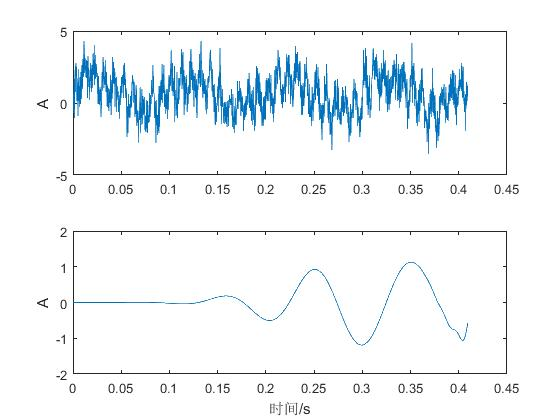图1.jpg (28.1 KB, 下载次数: 6)图12017-8-14 15:04 上传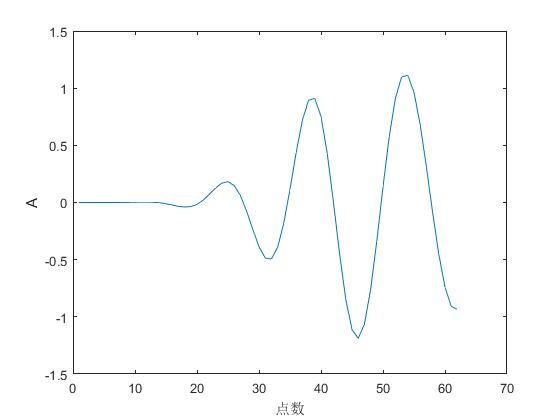图2.jpg (14.35 KB, 下载次数: 4)图22017-8-14 15:04 上传
展开全文• 滤波器按照频带划分可以分为：低通滤波器(LPF)、高通滤波器(HPF)、带通滤波器(BPF)、带阻滤波器(BEF)。其中射频天线领域主要采用带通滤波器(BPF)。 2 带通滤波器(BPF) ①：RF BPF：从天线中取出期望频带的有效信号...
一、简介
1 滤波器
滤波器按照频带划分可以分为：低通滤波器(LPF)、高通滤波器(HPF)、带通滤波器(BPF)、带阻滤波器(BEF)。其中射频天线领域主要采用带通滤波器(BPF)。
2 带通滤波器(BPF)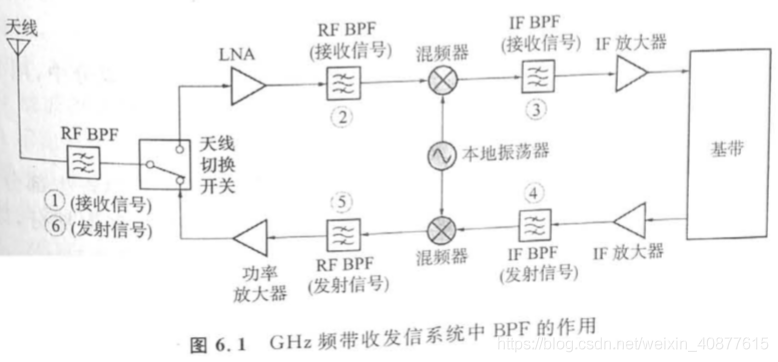①：RF BPF：从天线中取出期望频带的有效信号，滤除不需要频带的电磁波信号和噪声。
②：RF BPF：用下级混频器(降频混频器)进行频率转换时，防止在IF频带中引入干扰信号，用BPF将不期望频率成分滤除。
③：IF BPF：从混频器进行频率转换所产生的许多频率成分中，用BPF只取出期望的IF信号，也具有限制接收信号频带的作用。
④：IF BPF：用混频器(升频转换器)进行频率转换时，用BPF防止在RF频带中引入干扰信号，也具有限制发射信号频带的作用。
⑤：RF BPF：从混频器进行频率转换所产生的许多频率成分中，用BPF只取出期望的RF信号。
⑥：RF BPF：用BPF除去功率放大器放大时失真所产生的寄生部分，以及大功率信号的输入在天线开关时所产生的寄生部分，以免寄生成分通过天线发射出去。
3 带通滤波器(BPF)选择：
(1)对于低频电路，选择LC滤波器。LC滤波器通常用于基频领域。
(2)对于100MHz~1000MH频率范围，选择SAW滤波器。SAW滤波器通常用于IF BPF领域。
(3)对于2GHz频率电路，选择介质滤波器。介质滤波器通常用于RF BPF领域。
4 带通滤波器(BPF)详解：
核心思想：BPF基本上是谐振电路。谐振电路分为串联谐振电路和并联谐振电路，对于串联谐振电路，若L和C无损耗，则在谐振频率时阻抗变为零；对于并联谐振电路，谐振频率时阻抗变为无穷大。对于串联谐振电路可知，L越大，衰减特性越陡；对于并联谐振电路可知，L越小C越大，衰减特性越陡。
谐振电路的谐振频率：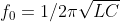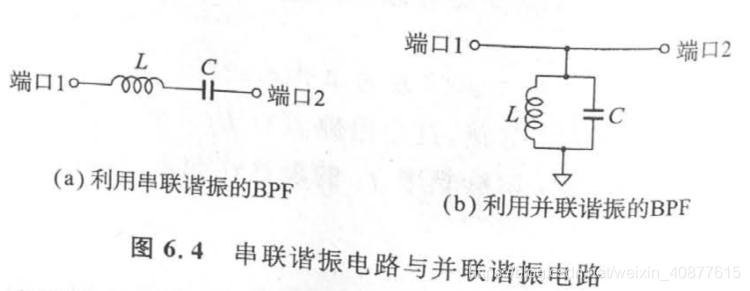串联谐振与并联谐振的组合电路：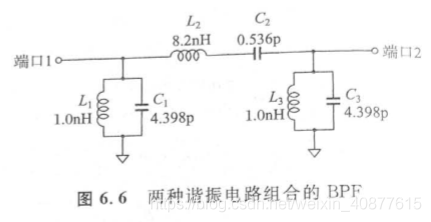5 介质滤波器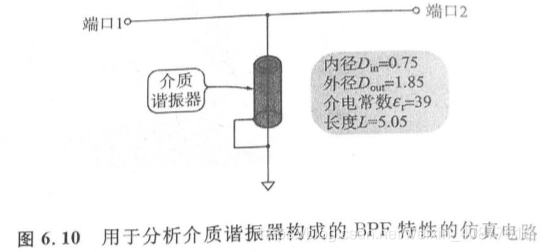六 微带线制作滤波器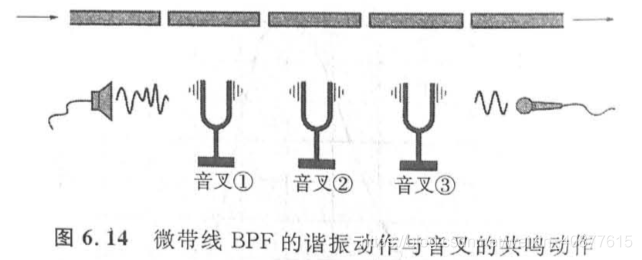二、源代码
clear all;
file_name = 'Data1.txt';    % 文件名
fid =fopen(file_name,'r');  %打开文件
h=fgets(fid);               %去掉第一行；
C=textscan(fid,'%*f%f%f%f%f');%去掉第一列；并将其他行列写入C
fclose(fid); %关闭文件
Data=[C{1}';C{2}';C{3}';C{4}'];%将C中的数据取出放入数组Data1
fid =fopen(['new_' file_name],'w'); %打开新文件夹
fprintf(fid,'%-.10e    %-.10e   %-.10e   %-.10e\r\n  ',Data); %将取出的数据放入新文件夹，纯数据
fclose(fid);%关闭新文件夹
t=['new_' file_name];

x1=newData(:,1);
x2=newData(:,2);
x3=newData(:,3);
x4=newData(:,4);

figure(1);
subplot(4,1,1)
plot(x1);
axis([0 512 0.5 1.7]);grid on;
% figure;
subplot(4,1,2)
plot(x2);
axis([0 512 0.5 1.7]);grid on;
% figure;
subplot(4,1,3)
plot(x3);
axis([0 512 0.5 1.7]);grid on;
% figure;
subplot(4,1,4)
plot(x4);
axis([0 512 0.5 1.7]);grid on;
N=512;
M=1; %调节因子

fs=1000;
%数据点数
%时间序列
n=0:N-1;
f=n*fs/N;    %频率序列
%%%%%%%%%%%%%%%%%%%%%%%%%%%%%%%%%%%%%%%%%%%%%%%
%信号
figure(2);

y1=fft(x1,N);    %对信号进行快速Fourier变换
mag1=abs(y1);     %求得Fourier变换后的振幅
subplot(4,1,1) ,plot(f(1:N/2),mag1(1:N/2));
axis([0 500 0 20]);grid on;

y2=fft(x2,N);    %对信号进行快速Fourier变换
mag2=abs(y2);    %求得Fourier变换后的振幅
subplot(4,1,2), plot(f(1:N/2),mag2(1:N/2));
axis([0 500 0 20]);grid on;

y3=fft(x3,N);    %对信号进行快速Fourier变换
mag3=abs(y3);     %求得Fourier变换后的振幅
subplot(4,1,3) ,plot(f(1:N/2),mag3(1:N/2));
axis([0 500 0 20]);grid on;

y4=fft(x4,N);    %对信号进行快速Fourier变换
mag4=abs(y4);     %求得Fourier变换后的振幅
subplot(4,1,4), plot(f(1:N/2),mag4(1:N/2));
axis([0 500 0 20]);grid on;
%=====%part3%===========%带通滤波器%========%
Wp = [50 150]/1000; Ws = [10 500]/1000;
Rp = 1; Rs =50;
[n,Wn] = buttord(Wp,Ws,Rp,Rs)
[b1,a1] = butter(n,Wn);
figure(3)
freqz(b1,a1)
title('n=4 Butterworth Bandpass Filter');
%=====%part3%===========%带通滤波器%========%
%=====%part4%===========%50hz陷波器%=======%
Wp = [10 500]/1000; Ws = [49 51]/1000;
Rp = 1; Rs = 50;
[n,Wn] = buttord(Wp,Ws,Rp,Rs)
[b2,a2] = butter(n,Wn,'stop');
figure(4)
freqz(b2,a2)
title('n=2 Butterworth Bandstop Filter');
%=====%part4%===========%50hz陷波器%=======%
%=====%part5%=============%数字滤波%========%
x1=filter(b1,a1,x1);
x1=filter(b2,a2,x1);
x2=filter(b1,a1,x2);
x2=filter(b2,a2,x2);
x3=filter(b1,a1,x3);
x3=filter(b2,a2,x3);
x4=filter(b1,a1,x4);
x4=filter(b2,a2,x4);

figure(5);
subplot(4,1,1)
plot(x1);grid on;
axis([0 512 -0.5 0.5])
subplot(4,1,2)
plot(x2);grid on;
axis([0 512 -0.5 0.5])
subplot(4,1,3)
plot(x3);grid on;
axis([0 512 -0.5 0.5])
subplot(4,1,4)
plot(x4);grid on;
axis([0 512 -0.5 0.5])

%=====%part5%=============%数字滤波%========%
figure(6);
y1=fft(x1,N);    %对信号进行快速Fourier变换
mag1=abs(y1);     %求得Fourier变换后的振幅
subplot(4,1,1) ,plot(f(1:N/2),mag1(1:N/2));
axis([0 500 0 11]);
grid on;

y2=fft(x2,N);    %对信号进行快速Fourier变换
mag2=abs(y2);    %求得Fourier变换后的振幅
subplot(4,1,2), plot(f(1:N/2),mag2(1:N/2));
axis([0 500 0 10]);
grid on;

y3=fft(x3,N);    %对信号进行快速Fourier变换
mag3=abs(y3);     %求得Fourier变换后的振幅
subplot(4,1,3) ,plot(f(1:N/2),mag3(1:N/2));
axis([0 500 0 10]);
grid on;

y4=fft(x4,N);    %对信号进行快速Fourier变换
mag4=abs(y4);     %求得Fourier变换后的振幅
subplot(4,1,4), plot(f(1:N/2),mag4(1:N/2));
axis([0 500 0 10]);
grid on;
%=====%part6%=============%验证效果%========%
iemg1=sum(abs(x1))/length(x1);
iemg2=sum(abs(x2))/length(x2);
iemg3=sum(abs(x3))/length(x3);
iemg4=sum(abs(x4))/length(x4);

三、运行结果四、备注
版本：2014a


展开全文• ## matlab实现带通滤波

万次阅读 2020-07-22 15:19:25
matlab给出的代码如下：[n,Wn] = buttord(Wp,Ws,Rp,Rs) 首先要先理解一个带通滤波器的幅频特性图中，分为通带、阻带、过渡带三大部分。 通带截止频率和阻带截止频率对这三部分进行划分，二者之间的部分即为过渡带...
以带通滤波器——巴特沃斯滤波器为例

matlab给出的代码如下：[n,Wn] = buttord(Wp,Ws,Rp,Rs)

首先要先理解一个带通滤波器的幅频特性图中，分为通带、阻带、过渡带三大部分。

通带截止频率和阻带截止频率对这三部分进行划分，二者之间的部分即为过渡带。

一.如何设计带通滤波器?

1.Wp,Ws分别是通带频率和阻带截止频率

这两个频率是指同一侧的通带和阻带频率，比如设计一个40Hz~100Hz的带通滤波器

Wp1=40Hz,Wp2=100Hz,代表Wp1~Wp2之间是通带

Ws1=20Hz,Ws2=120Hz,代表0~20Hz和120Hz~正无穷为阻带

过渡带宽度=阻带边缘频率-通带边缘频率（高频的一侧）=Ws2-Wp2=20Hz

2.Rp,Rs是描述通带、阻带幅值的两个重要参量

Rp是描述通带波纹（起伏程度）的一个参量，通带纹波是指在滤波器的频响中通带的最大幅值和最小幅值之间的差值，正常的纹波一般小于1db。

通带波纹当然越小越好，这样通带内频率的幅度都基本稳定在单倍幅度上，因此Rp是允许的通带波纹的最大值。

Rs是描述阻带衰减的一个参量

阻带衰减越大越好，衰减越大代表对不想要的信号频率成分的滤除效果越好，因此Rs是允许的需要达到的阻带衰减的最小值。

3.n,Wn是设计得到滤波器的阶数和截止频率

通道滤波器的设计代码例程如下：

Wp = [40 100]/500;
Ws = [20 120]/500;
Rp = 3;
Rs = 40;
[n,Wn] = buttord(Wp,Ws,Rp,Rs)

上述在Wp,Ws的赋值过程中进行了归一化，因此run之后得到的Wn的值也是归一化过的

n = 12
Wn = 0.0799    0.2002

二.如何绘制带通滤波器的幅频响应?

可以先调用butter()函数返回巴特沃斯滤波器传递函数的零极点系数和增益。

[z,p,k] = butter(n,Wn);
sos = zp2sos(z,p,k);

freqz(sos,512,1000)
title(sprintf('n = %d Butterworth Lowpass Filter',n))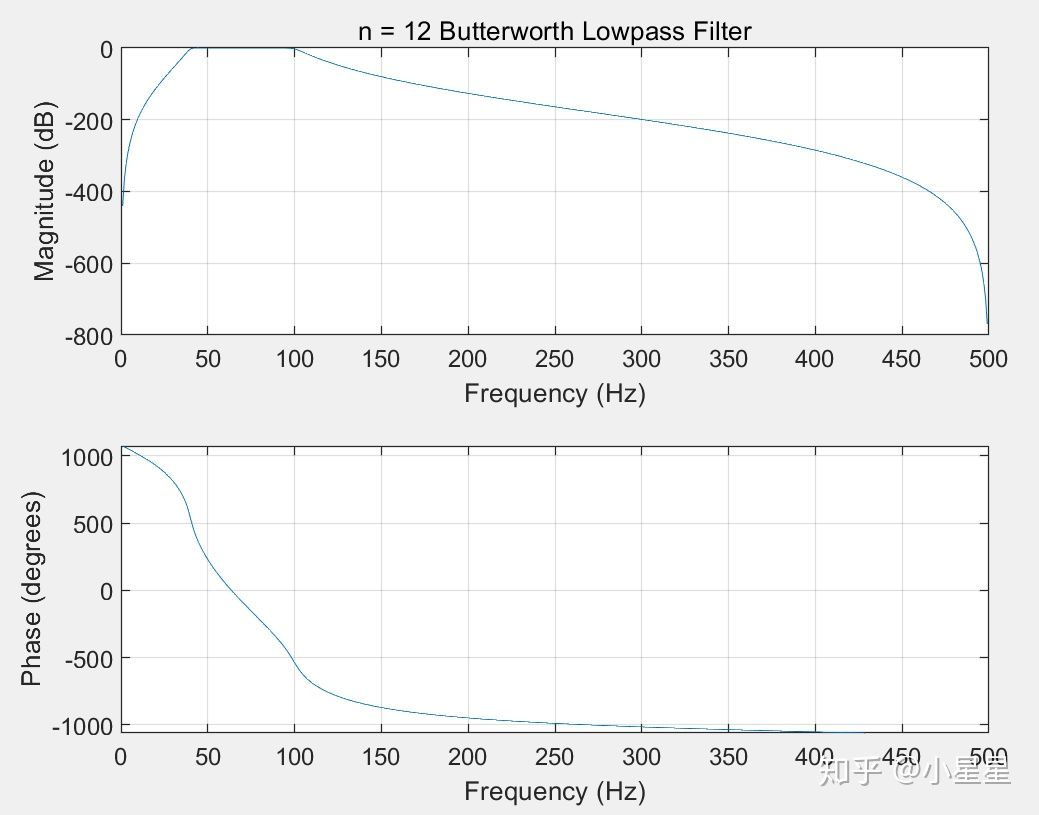三.如何使用带通滤波器对一组数据进行滤波

y = filter(b,a,x) 

利用分子和分母系数 b 和 a 定义的有理传递函数对输入数据 x 进行滤波。

https://zhidao.baidu.com/question/423138525.html

四.如何合理确定滤波器的参数？

我们已知所需要的通带截止频率，阻带截止频率，可以通过一个便捷的方式由matlab直接确定滤波器的参数。输入fdatool命令,具体方法可参见以下博客。

https://blog.csdn.net/qq_42839007/article/details/104184920

https://wenku.baidu.com/view/6bb31c23dcccda38376baf1ffc4ffe473368fd82.html

滤波的阶数
滤波器的阶数，就是滤波几次的意思，一阶，就是对谐波过滤一次，滤波器的阶数越高，滤波效果越好，但是，成本也会成倍的增加，因此，选择合适的阶数是非常重要的。


展开全文信号处理 数字通信 iir滤波器
• ylabel(‘幅度/dB‘) title(‘幅频特性‘) subplot(2,2,2) plot(angle(hk)) xlabel(‘频率/Hz‘) ylabel(‘相位‘) title(‘相频特性‘) 程序运行结果： 巴特沃斯模拟带阻滤波器阶数：Nb=4 2、带通滤波器的设计 带通...
• 理想带通使用如下函数，dim是维度，如果input是一维，则dim=1% FILTERED = ideal_bandpassing(INPUT,DIM,WL,WH,SAMPLINGRATE)%% Apply ideal band pass filter on INPUT along dimension DIM.%% WL: lower cutoff ...
• ## matlab理想带通滤波

万次阅读 2017-10-20 20:03:19
理想带通使用如下函数，dim是维度，如果input是一维，则dim=1 % FILTERED = ideal_bandpassing(INPUT,DIM,WL,WH,SAMPLINGRATE) % % Apply ideal band pass filter on INPUT along dimension DIM. % % WL: lower ...
• 它使用频域中的矩形窗口对时间序列进行带通滤波，“无需外部工具箱”。 下面给出了一个示例代码，用于过滤 200~300 Hz 通带中的时间序列： Fs = 1000； t = linspace(0,1,Fs); x = cos(2*pi*100*t)+0.5*randn(size(t...
• ## 带通滤波matlab

千次阅读 2019-01-26 20:02:00
巴特沃斯： 1、带阻滤波器设计 带阻滤波器指标： 阻带上边界频率：5Kz； 阻带下边界频率：7Kz；...巴特沃斯模拟带通滤波器阶数：N=5 转载于:https://www.cnblogs.com/hjj-fighting/p/10324768.html
• 动态光散射激光自混频信号的带通滤波处理技术，沈建琪，王华睿，采用激光自混频技术进行动态光散射纳米颗粒测量，并提出利用带通滤波技术对动态散射光信号进行处理。对自相关函数法、功率谱密度
• 数字图像处理三级项目 咼通低通带通滤波命 摘要 在图像处理的过程中消除图像的噪声干扰是一个非常重要的问题利用 matlab软件,采用频域滤波的方式,对图像进行低通和高通滤波处理低通滤波 是要保留图像中的低频分量而...
• bandpass带通滤波详解基本使用适用范围注意事项原理解释 基本使用 先上代码，下面是带通滤波器在matlab中的使用 function [ pc ] = m_bandpass( pc ) freq = [0.1 0.5]; fs= 50; % Fa=Fs/2，Fa是分析频率 ...
• 一个混合正弦信号包含5Hz，15Hz、30Hz的3种频率信号，现要求设计适当的滤波器，保留15Hz频率分量信号，给定采样频率100Hz。
• 对含有周期性噪声图像进行高斯带通滤波，基于ｍａｔｌａｂ，程序核心无调用内部函数，效果良好，供大家参考
• 标准实用文案 数字图像处理三级项目 高通 低通带通滤波器 文档 标准实用文案 摘要 在图像处理的过程中 ,消除图像的噪声干扰是一个非常重要的问题利用 matlab 软件 ,采用频域滤波的方式 ,对图像进行低通和高通滤波...
• 数字图像处理三级项目 高通低通带通滤波器 摘要 在图像处理的过程中 , 消除图像的噪声干扰是一个非常重要的问题利用 matlab 软件 , 采用频域滤波的方式 , 对图像进行低通和高通滤波处理低通滤波 是要保留图像中的...
• 巴特沃斯带通滤波器这个简单的函数是为我的数字图像处理课程编写的在东地中海大学任教副. 哈桑·德米雷尔（Hasan Demirel）教授2010-2011 Spring学期完整报告： ...
• (ii) 带通滤波：提高 SNR，尤其是在系统谐振附近执行时(iii) 平方包络谱：允许检测以特定循环频率的大分量为特征的（伪）循环平稳贡献此功能与简单的演示一起提供，它与 Octave 完全兼容。 参考文献：Borghesani P...
• 最近有一个需求，在C#中实现FIR滤波，网上查了些资料感觉FIR滤波使用的还算比较多，相关的原理也比较简单。参考下面在Python环境中实现FIR的博客，在C#的环境中实现了一遍。 ...C#
• ## PR谐振，带通滤波

千次阅读 2016-04-11 17:05:24
PR比例谐振传递函数也是一个带通滤波，不过通带接近0，不能拿来滤波，可以用准谐振，它类似于一个二阶带通滤波！ 离散状态方程，可以转换为Z域传递函数；连续状态方程，转换为S域传递函数；S与Z域传递函数...filter
• ## mtalab-fir1 带通滤波

千次阅读 2015-07-08 14:31:54
function [ res ] = bandPassSef(data,Fs,Fc1,Fc2) %BANDPASSSEF Summary of this function goes here % Detailed explanation goes here %Fs = 48000; % Sampling Frequency N = 100; % Order
• 获取输入图像，根据上下空间频率阈值修改其频域，并返回过滤后的图像。 这是一个“硬”滤波器，因为阈值频率内的频域中的所有值都乘以 1，阈值之外的所有值都乘以 0。 该程序可用于隔离特定频率；...
• matlab带通滤波器，可以很好的实现带通滤波
• 提供了matlab 数据滤波，低通滤波，带通滤波，谱分析相关算法及实验数据，可用于故障诊断和分析
• 本套课程适合对象1 想入门matlab信号处理的初学者；2 想深入通过信号处理案例来实战的中级学者；本套课程特色1 2018年最新录制高清加完整演示代码；...滤波包括高通滤波、低通滤波、带通滤波、维纳滤波、卡尔曼滤波...
• 在前一段时间遇到了一个MATLAB带通滤波方面的问题，滤波后首尾段数据都出现异常状况，然后就开始疯狂的查资料，但是这方面的资料说实话确实很少，尤其是这种滤波器性能导致的问题，后来偶然的巧合下，在MATLAB中文...异常处理
• %带通滤波 %使用注意事项：通带或阻带的截止频率与采样率的选取范围是不能超过采样率的一半 %即，f1,f3,fs1,fsh,的值小于 Fs/2 %x:需要带通滤波的序列 % f 1：通带左边界 % f 3：通带右边界 % fs1：衰减截止左边界 %......

# matlab带通滤波matlab 订阅